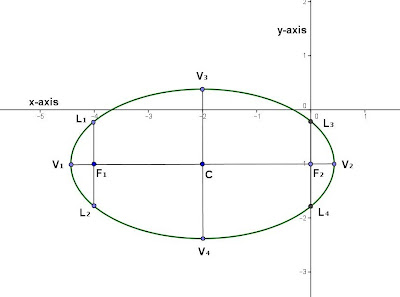## Thursday, January 3, 2013

### Graphical Sketch - Ellipse

Category: Analytic Geometry, Algebra

"Published in Newark, California, USA"

Find the center, foci, and semi-axes for the ellipse and sketch the graph

Solution:

From the given equation above, the curve is ellipse because both x2 and y2 are positive but their coefficients are different.  The general equation of an ellipse must be simplified in standard form in order to get its center, foci, and semi-axes as follows

Group the equation according to their variables and transpose the coefficient to the right side of the equation

Complete the square of each group

Divide both sides of the equation by 6

The above equation is now simplified in standard form. Since the denominator at x group is greater than the denominator at y group, then the major axis is parallel to x-axis.

To solve for the coordinates of the center:

Equate x + 2 = 0                                     Equate y + 1 = 0
x = -2                                                      y = -1

Therefore, the coordinates of the center is C(-2, -1).

Next, solve for the value of semi-major axis

Therefore, the coordinates of the ends of major axis are V1(-4.4495, -1) and V2(0.4495, -1).

Next, solve for the value of semi-minor axis

Therefore, the coordinates of the ends of minor axis are V3(-2, 0.4142) and V4(-2, -2.4142)

Next, solve for the distance of a focus to the center

Therefore, the coordinates of the foci are F1(-4, -1) and F2(0, -1)

Next, solve for the length of a semi-latus rectum

Add and subtract this value to the y values of foci in order to get the coordinates of the ends of latera recta. Therefore, the coordinates of the ends of latera recta are L1(-4, 0.1835), L2(-4, -1.8165), L3(0, 0.1835), and L4(0, -1.8165)

Since we know the coordinates of the center, ends of major axis, ends of minor axis, foci, and ends of latera recta, then we can sketch the graph of an ellipse as followsPhoto by Math Principles in Everyday Life

## Wednesday, January 2, 2013

### Arithmetic Progression - Salary Increase

Category: Algebra

"Published in Newark, California, USA"

Noel was hired by a local petroleum refinery at a starting salary of ₱ 40,000 a month. If the company gives him a ₱ 30,000 increase in his annual salary every year, how much would he be getting at the start of his 10th year with the company? If he regularly saves 10% of his monthly salary, what will be his total savings at the start of the 10th year?

Solution:

From the analysis of a given word problem, this is an application of arithmetic progression because there's a common difference in the sequence which is ₱ 30,000 per year, the amount of annual salary increase of Noel.

The initial annual salary of Noel = ₱ 40,000 (12) = ₱ 480,000.

First, the nth term of an Arithmetic Progression is give by the formula

where

a1first term of an arithmetic progression

an = last term of an arithmetic progression

n = nth term of an arithmetic progression

d = common difference of an arithmetic progression

In the given word problem,

an = amount of Noel's salary at the end of his 10th year with the company

a1initial salary of Noel which is ₱ 480,000

n = number of years in working with the company which is 10 years

d = amount of annual salary increase of Noel which is ₱ 30,000

Substitute the given items to the above equation in order to get the amount of Noel's salary at the end of his 10th year with the company as follows

Therefore, the amount of Noel's salary at the end of his 10th year with the company is ₱ 750,000. We can calculate his monthly salary at the start of his 10th year with the company as follows

Therefore, his monthly salary at the start of his 10th year with the company is ₱ 62,500.

Next, the Sum of an Arithmetic Progression is given by the formula

where

Sn = sum of the terms of an arithmetic progression from a1 to an

a1 = first term of an arithmetic progression

n = nth term of an arithmetic progression

d = common difference of an arithmetic progression

In the given word problem,

a1 = initial salary of Noel which is ₱ 480,000

S9 = sum of Noel's salary at the start of his 10th year with the company. In this calculation, we will use 9 years because the problem says "at the start of his 10th year".

n = number of years in working with the company which is 10 years. In this calculation, we will use 9 years because the problem says "at the start of his 10th year".

d = amount of annual salary increase of Noel which is ₱ 30,000

Substitute the given items to the above equation in order to get the sum of Noel's salary at the start of his 10th year with the company as follows

Therefore, his total savings at the start of his 10th year with the company is

Savings = 0.10 S9

Savings = 0.10 (₱ 5,400,000)

Savings = ₱ 540,000

Note: The symbol, ₱ is Philippine Pesos.